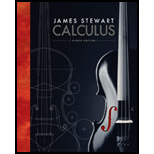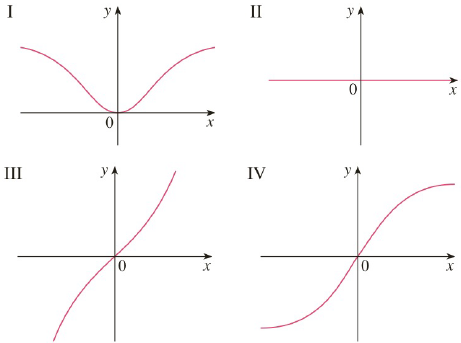# Match the differential equations with the solution graphs labeled I–IV. Give reasons for your choices. (a) y ′ = 1 + x 2 + y 2 (b) y ′ = x e − x 2 − y 2 (a) y ′ = 1 1 + e x 2 + y 2 (a) y ′ = sin ( x y ) cos ( x y )### Calculus (MindTap Course List)

8th Edition
James Stewart
Publisher: Cengage Learning
ISBN: 9781285740621

#### Solutions

Chapter
Section### Calculus (MindTap Course List)

8th Edition
James Stewart
Publisher: Cengage Learning
ISBN: 9781285740621
Chapter 9.1, Problem 13E
Textbook Problem
302 views

## Match the differential equations with the solution graphs labeled I–IV. Give reasons for your choices.(a) y ′ = 1 + x 2 + y 2 (b) y ′ = x e − x 2 − y 2 (a) y ′ = 1 1 + e x 2 + y 2 (a) y ′ = sin ( x y ) cos ( x y )To determine

To match:

The differential equation with the solution graphs and to give the reasons for choices.

### Explanation of Solution

1) Concept:

i. From the graph of function see the nature of function.

ii. P is increasing if dPdt>0 and decreasing if dPdt<0

2) Given:

The differential equation y'=1+x2+y2

And the solution graphs are,

3) Calculation:

The given differential equation is

y'=1+x2+y2

And the graph of functions is

Consider y'=1+x2+y2

Notice that, y'=1+x2+y21 for all values of x & y and as x increases y' is also increases, as x, y'

From the above graph see that only graph with labelled III is satisfactory with this condition.

Therefore, the differential equation y'=1+x2+y2 matches with the solution graph labelled  III, because it is reasonable with the condition, as x, y'

Conclusion:

The differential equation y'=1+x2+y2 matches with the solution graph labelled  III, because it is reasonable with the condition, as x, y'

To match:

The differential equation with the solution graphs and to give the reasons for choices.

Solution:

The differential equation y'=xe-x2-y2 matches with the solution graph labelled  I, because it is only graph reasonable with the condition, y'>0 for x>0 and y'<0 if  x<0

1) Concept:

i. From the graph of function see the nature of function.

ii. P is increasing if dPdt>0 and decreasing if dPdt<0

2) Given:

The differential equation y'=xe-x2-y2

And the solution graphs

3) Calculation:

The given differential equation is

y'=xe-x2-y2

And the graphs of functions are

Consider y'=xe-x2-y2

Notice that, y'=xe-x2-y2>0 for x>0 and y'=xe-x2-y2<0 if  x<0

From the above graph see that only function with negative tangent slope ( that is decreasing) when x<0 and positive tangent slope (increasing) when x>0 is function in graph labelled I

Therefore, the graph with labelled I is satisfactory with this condition.

Therefore, the differential equation y'=xe-x2-y2 matches with the solution graph labelled  I, because it is only graph reasonable with the condition, y'>0 for x>0 and y'<0 if  x<0

Conclusion:

The differential equation y'=xe-x2-y2 matches with the solution graph labelled  I, because it is only graph reasonable with the condition, y'>0 for x>0 and y'<0 if  x<0

To match:

The differential equation with the solution graphs and to give the reasons for choices.

Solution:

The differential equation y'=11+ex2+y2  matches with the solution graph labelled  IV, because it is only graph reasonable with the condition, y'>0 for x and y'0 as  x

1) Concept:

i. From the graph of function see the nature of function.

ii. P is increasing if  dPdt>0 and decreasing if  dPdt<0

2) Given:

The differential equation y'=11+ex2+y2

And the solution graphs are

### Still sussing out bartleby?

Check out a sample textbook solution.

See a sample solution

#### The Solution to Your Study Problems

Bartleby provides explanations to thousands of textbook problems written by our experts, many with advanced degrees!

Get Started

Find more solutions based on key concepts
Evaluate the integral. 28. tan5xsec3xdx

Calculus: Early Transcendentals

SPENDING on Equipment and Software As the United States continues to recover slowly from the Great Recession, s...

Applied Calculus for the Managerial, Life, and Social Sciences: A Brief Approach

The polar form for the graph at the right is:

Study Guide for Stewart's Multivariable Calculus, 8th

356dx= a) 2 b) 8 c) 6 d) 12

Study Guide for Stewart's Single Variable Calculus: Early Transcendentals, 8th

Consider a sample with data values of 10, 20, 21, 17, 16, and 12. Compute the mean and median.

Statistics for Business & Economics, Revised (MindTap Course List)

Write x2+12x+1 in the form a(xp)2+q.

Functions and Change: A Modeling Approach to College Algebra (MindTap Course List)

55. A large consumer goods company ran a television advertisement for one of its soap products. On the basis of...

Modern Business Statistics with Microsoft Office Excel (with XLSTAT Education Edition Printed Access Card) (MindTap Course List)

If o(E)=a:b, find o(E).

Mathematics: A Practical Odyssey

In Problems 118 use Definition 7.1.1 to find f(t). 5. f(t)={sint,0t0,t

A First Course in Differential Equations with Modeling Applications (MindTap Course List)